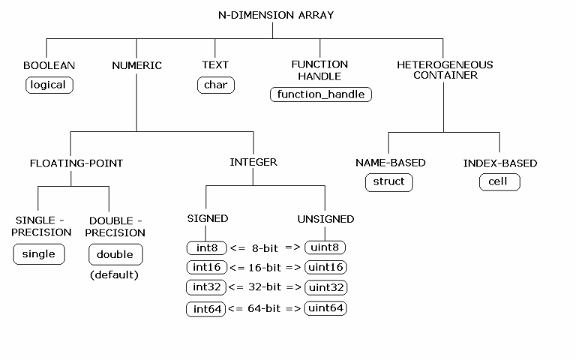## Data Conversion with Java and MATLAB Types

### Working with MATLAB Data Types

There are many data types that you can work with in MATLAB®. Each of these data types is in the form of a matrix or array. You can build matrices and arrays of floating-point and integer data, characters and strings, and logical true and false states. Structures and cell arrays provide a way to store dissimilar types of data in the same array.

All of the fundamental MATLAB classes are circled in the diagram Fundamental MATLAB Data Types.

The Java® client follows Java-MATLAB-Interface (JMI) rules for data marshaling. It expands those rules for scalar Java boxed types, allowing auto-boxing and un-boxing, which JMI does not support.

Note

Function Handles are not supported by MATLAB Production Server™.

Fundamental MATLAB Data TypesThe expected conversion results for Java to MATLAB types are listed in Conversion of Java Types to MATLAB Types. The expected conversion results for MATLAB to Java types are listed in Conversion of MATLAB Types to Java Types.

### Scalar Numeric Type Coercion

Scalar numeric MATLAB types can be assigned to multiple Java numeric types as long as there is no loss of data or precision.

The main exception to this rule is that MATLAB `double` scalar data can be mapped into any Java numeric type. Because `double` is the default numeric type in MATLAB, this exception provides more flexibility to the users of MATLAB Production Server Java client API.

MATLAB to Java Numeric Type Compatibility describes the type compatibility for scalar numeric coercion.

MATLAB to Java Numeric Type Compatibility

MATLAB TypeJava Types
`uint8``short`, `int`, `long`, `float`, `double`
`int8``short`, `int`, `long`, `float`, `double`
`uint16``int`, `long`, `float`, `double`
`int16``int`, `long`, `float`, `double`
`uint32``long`, `float`, `double`
`int32``long`, `float`, `double`
`uint64``float`, `double`
`int64``float`, `double`
`single``double`
`double``byte`, `short`, `int`, `long`, `float`

### Dimensionality in Java and MATLAB Data Types

In MATLAB, dimensionality is an attribute of the fundamental types and does not add to the number of types as it does in Java.

In Java, `double`, `double[]` and `double[][][]` are three different data types. In MATLAB, there is only a `double` data type and possibly a scalar instance, a vector instance, or a multi-dimensional instance.

Java SignatureValue Returned from MATLAB
`double[][][] foo() ``ones(1,2,3)`

#### Dimension Coercion

How you define your MATLAB function and corresponding Java method signature determines if your output data will be coerced, using padding or truncation.

This coercion is automatically performed for you. This section describes the rules followed for padding and truncation.

### Empty (Zero) Dimensions

Passing arrays of zero (`0`) dimensions (sometimes called empties) results in an empty matrix from MATLAB.

Java SignatureValue Returned from MATLAB
`double[] foo() ``[]`

### Boxed Types

Boxed Types are used to wrap opaque C structures.

Java client will perform primitive to boxed type conversion if boxed types are used as return types in the Java method signature.

Java SignatureValue Returned from MATLAB
`Double foo()``1.0`

For example, the following method signatures work interchangeably:

```double[] foo(); Double[] foo(); double[][][] foo(); Double[][][] foo(); ```

### Signed and Unsigned Types in Java and MATLAB Data Types

Numeric classes in MATLAB include signed and unsigned integers. Java does not have unsigned types.